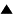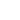# The Physics of Evolution

From the viewpoint of a physicist, evolution can be seen as a search for stability. Consider a system of atoms in the framework of non-relativistic quantum mechanics and statistics. Its configuration is a set of values of all generalized coordinates of the system, e.g., the coordinates of all atomic nuclei and electrons. The system is described by Schrödinger's equation which includes the potential energy of the system as a function of its configuration. This function can be visualized as a distribution in the n-dimensional configuration space, where n is the number of the degrees of freedom. The solutions of the Schrödinger equation for a given total energy E of the system constitute the total set of all possible quantum states of the system. When we deal with a macroscopic system, though, it is never completely isolated, but constantly exchanges energy with its environment, so there is an interval DE of energy within which the exact energy of the system varies. Let the total set of states with energies around E and inside DE be S. The system jumps randomly within this set from one state to another. The logarithm of the number of states in S is the entropy of the system: H = ln |S|.

We also can see the state of a macroscopic system in the quasi-classical approximation as a point moving in its phase space. The phase space has twice as many coordinate axes as the configuration space: for each generalized coordinate it includes the corresponding generalized impulse. The states available for the system make a surface of a given energy E, or, more precisely, the space between the surfaces for E and E+DE. The volume of this space measured in the units of the Plank's constant h is the same number of quantum states |S| as above. It defines the entropy of the system.

A quantum system tends to find itself in a configuration which has the minimum of potential energy. But the potential energy of a macroscopic system is an extremely complex and irregular function. It has a stupefying combinatorial number of local minima and maxima. If the system finds itself in a local minimum of energy, it must overcome a potential barrier in order to leap to another minimum. The probability of such a leap includes the factor e^{-b/T}, where b is the height of the barrier, and T is the temperature of the system, i.e. the average energy per one degree of freedom. Hence if the barrier is much greater than T, the probability of jumping over it is exceedingly small.

Imagine a potential energy function which looks like a crater on the moon: an area C_1 surrounded by a pretty high (as compared with the temperature T? circular ridge. Let the phase space volume corresponding to C_1 be S1. It is a subset of the total set of states S, so S1 \subset S. Accordingly, as long as the system stays in S1 its entropy H1 is less than for the system free to be found in any state of S: H1 < H.

Now the following fundamental fact is in order: given two quantum states, s1 and s2, the probability of transition from s1 to s2 is the same as that of the inverse transition from s2 to s1. If at some time the system is found in the state s1 \in S1, it may stay there until a transition to some state s2 occurs, which is not in S1: s2 \in S2 = (S - S1). But the probability that it will get back from S2 to S1 is even much less; for macroscopic phenomena it is so small that it is, in fact, impossible. Indeed, let the probability rate of a transition between the states of S1 and S2 be of the order of magnitude p. Then the probability of jumping from (any state in) S1 to (any state in) S2 is p|S2|, while the probability of the inverse transition is p|S1|. Recall now that S1 results from a certain constraint on S. The properties of combinatorial numbers are such that if a constraint is removed, the number of combinations increases at a mind-boggling rate. Thus |S| is not just greater than |S1|, but many, many times greater. Hence |S2| is also many times greater than |S1|. The probability of returning to S1 will be less than that of escaping from it by the factor:

[ |S1| / |S2| \approx |S1| / |S| = e^{-(H-H1)} ]

For macroscopic phenomena the difference between the entropies will be macroscopic, and the exponent vanishing.

Hence the law of the growth of entropy. A system will not jump from the larger set S to a smaller set S1. When a system changes its macroscopic state, its entropy can only increase.

In this light let us look at stability. As long as the system stays in the state S1, it preserves its identity. But sooner or later, under the influence of cosmic radiation, or just an especially big fluctuation of thermal energy, a quantum leap takes place and the system is in S2. Some part of its organization, defined as compliance with some specified constraint, is lost. The entropy went up. How can we bring the system back to S1?

The answer is: we need a certain amount of energy to overcome potential barriers. But there is an additional requirement to that energy: it must belong to a single agent, or, maybe, to a very few agents. An agent in this context is a force or forces associated with one degree of freedom, or a few degrees of freedom, between which there is a strong interaction (note: even deterministic classical mechanics cannot do without speaking of freedom). A big system of atoms can be divided into some regions within which there is a significant interaction, while the interaction between the regions is weaker by orders of magnitude. The potential barriers of which we speak are regional. So are the corresponding degrees of freedom (generalized coordinates). A jump over a barrier changes equilibrium values of a few generalized coordinates. To make this jump the system must obtain an amount of energy comparable with the height of the barrier and concentrated on the coordinates which take part in the jump. In the language of agents, we need to pass to the agent of the jump the necessary amount of energy. Then it becomes possible to make a jump which amends the deteriorated organization, or creates it anew.

Given an amount of energy, we must ask an important question about it: is this energy concentrated on a single agent, or pulverized among a huge amount of nearly independent agents. The latter is thermal energy; the former is known in thermodynamics as free energy (freedom again!). It is only free energy which creates organization. Energy distributed between a great number of independent agents is useless for organization, because there is no force which could collect it into an amount sufficient for overcoming potential barriers, while the probability that this happens by chance is virtually non-existent.

So, we used a quantum of energy to overcome a potential barrier and create a desired regional configuration of atoms. When the point representing a configuration jumps from one side of the barrier to the other, its level of energy changes little, if at all. Then where does the energy we passed to the system go? In the last analysis, it dissipates between all the agents in the system, i.e. converts to the thermal form. If we want to have a stable system, such as a living system, there must be a way to get rid of this thermal energy, otherwise the temperature will raise higher and higher until rampant agents of thermal motion kill all organization around.

We come to the conclusion that if we want to see a stable or growing organization, there must be a relatively small number of agents which maintain the organization, passing to it in the process some energy, which later escapes the system as thermal energy. This flow of energy where it enters the system in a low-entropy form, i.e. vested in a few number of agents, and leaves it in a high-entropy thermal form, is essential for preserving organization.

It is often thought that the lower the energy of a system is, the more stable it is, but this is not accurate. A system may be in a state with relatively low energy but surrounded by low potential barrier. It will have much greater probability to jump some place than the same system in a state with a higher equilibrium energy, but surrounded by a high potential barrier. Stability is a feature of the configuration-energy function of the system. Theoretically, it is this function that is studied by cyberneticians and biologists. We are interested in the structure of the energy function of systems, the existence of local minima well protected by potential barriers. Evolution, and life itself, is the wandering of the system around local minima, in search of more and more protected configurations.

The forms of matter we see in nature are subsets of the total set of all possible configurations of any physical system. Stability of a form is measured by how long a configuration remains in its subset. There are two kinds of stability: that of dead and of living forms. They are different in the way the stability is achieved. A dead form is a subset of configurations enclosed by a high potential barrier at a low temperature, which makes the probability of getting out very small. A living form maintains stability by permanent self-repairing. For this it needs a flow of low-entropy energy, which it transforms into the high-entropy thermal form. There are different ways of self-repairing, including replication of living formations or their parts.

 HomeMetasystem Transition TheoryEvolutionary Theory Up Prev.Next Down